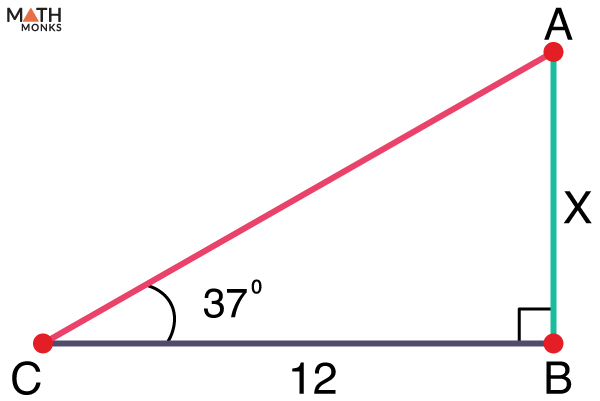# Tangent Ratio

The tangent ratio is one of the trigonometric ratios for right-angled triangles. It is the ratio of the opposite side to the adjacent side concerning an angle.

## Formula

Consider a right triangle ABC, where AC is the hypotenuse and AB and BC are the other two sides of a right triangle. Thus, for any angle θ in a right triangle,

${\tan \theta =\dfrac{Opposite side}{Adjacent side}}$

Precisely, ${\tan A=\dfrac{BC}{AB}}$ and ${\tan C=\dfrac{AB}{BC}}$

Thus, tangent ratios can be used to calculate the angles and sides of right-angle triangles, similar to the sine and cosine ratios. Furthermore, it is possible to find the tangent ratio given one angle of the right triangle other than the right angle.

Considering the tangent ratio ${\tan A=\dfrac{BC}{AB}}$, if the measure of angle A is known, it is possible to find the tangent ratio of angle A. Again, finding the tangent ratio is easy if one side and the hypotenuse are known. For example, if AC and BC are known, then by using the Pythagorean theorem, we get

${\left| AB\right| =\sqrt{\left| AC\right| ^{2}-\left| BC\right| ^{2}}}$

Thus, ${\tan A=\dfrac{\left| BC\right| }{\sqrt{\left| AC\right| ^{2}-\left| BC\right| ^{2}}}}$

## Finding the Tangent Ratio

Consider 3 right triangles with different side lengths but the same angle measuring θ. Let us now find the tangent ratios for all three triangles.

For the smaller triangle,

tan θ = ${\dfrac{4}{3}}$ = 1.34

For the medium triangle,

tan θ = ${\dfrac{8}{6}}$ = 1.34

For the large triangle,

tan θ = ${\dfrac{12}{9}}$ = 1.34

Thus, the tangent ratio remains the same regardless of the size of the right triangle. This specific ratio is the trigonometric ratio of the triangle for an acute angle θ, and it applies to similar triangles.

For example, tan 45° = 1

If one angle in a right triangle is 45°, the ratio of the length of the opposite leg to its adjacent leg is 1.

The tangent ratio is thus a function that takes different values depending on the angle measure. We can measure an angle in degrees or radians. It can also be calculated using a calculator.

## Solved Examples

Now that we learned the concept let us solve some problems.In the given right triangle PQR, find the tangent ratio of ∠P and ∠R

Solution:

tan P = ${\dfrac{QR}{QP}=\dfrac{12}{5}}$
tan R = ${\dfrac{PQ}{QR}=\dfrac{5}{12}}$Find the value of x in the given right triangle.

Solution:

As we know,
tan 37° = ${\dfrac{x}{12}}$
tan 37° ≈ 0.75
=> ${\dfrac{x}{12}}$ = 0.75
x = 9

Find the angle with a tangent ratio of 0.4877

Solution:

tan-1(0.4877) = 2525.99849161 ≈26 degrees

Find the angle with a tangent ratio of 0.9325.

Solution:

tan-1(0.9325) = 42.9995 ≈ 43 degrees